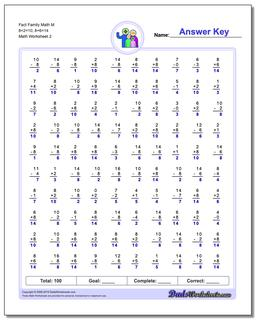# Math Worksheets: Fact Family Math: Fact Family Math: Fact Family Math M 8+2=10, 8+6=14 (Second Worksheet)## Fact Family Math M 8+2=10, 8+6=14 (Second Worksheet)

PropertyValue
DescriptionFact Family Math M 8+2=10, 8+6=14: These math worksheets have 100 addition and subtraction fact family problems and make for a challenging two minute test. (Second Worksheet)
Resource TypeWorksheet